##### Question

In: Statistics and Probability

# Of 200 girls 165 wanted to go to hill station for weekend. And of 210 186...

Of 200 girls 165 wanted to go to hill station for weekend. And of 210 186 boys preferred hill station. Using the .10 level of significance, can we conclude that there is a significant difference in the proportion of girls and the proportion of boys who preferred hill station?

(a) State the null hypothesis and the alternative hypothesis.

(b) What is the probability of a Type I error?

(c) Is this a one-tailed or a two-tailed test?

(d) What is the decision rule?

(e)What is the value of the test statistic?

(f)What is your decision regarding the null hypothesis?

(g) What is the p-value? Explain what it means in terms of this problem.

## Solutions

##### Expert Solution

Let the sample size of girls = n1 = 200
Number of girls who preferred hill station = X1 = 165
Proportion of girls who preferred hill station = p1 = X1/n1 = 165/200 = 0.825

Let the sample size of boys = n2 = 210
Number of boys who preferred hill station = X2 = 186
Proportion of boys who preferred hill station = p2 = X2/n2 = 186/210

(a) Null Hypothesis (H0) :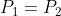,  i.e., there is no significant difference in the proportion of girls and the proportion of boys who preferred hill station.
versus,
Alternative Hypothesis (H1) :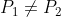,i.e., there is a significant difference in the proportion of girls and the proportion of boys who preferred hill station.

(b) Probability of type 1 error = P(rejecting H0 when H0 is true) = P(Z>mod(Zcal))=P(Z>1.7508)+P(Z<-1.7508) =0.07998
(c) This is a two tailed test since we are not testing for greater or lesser proportions. We are just testing for a significant difference irrespective of greater or lesser.

(d) The decision rule is we reject H0 if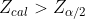(e) The test statistic is

Under H0: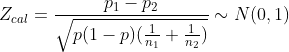where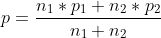Therefore,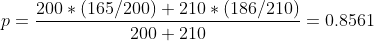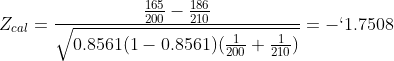(f) At 0.1 level of significance,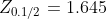Since modulus(Zcal) = 1.7508 >1.645, we reject H0 and conclude that there is a significant difference in the proportion of girls and the proportion of boys who preferred hill station.

(g) p-value is the probability of type 1 error which is 0.07998. Since, it is less than 0.1, we reject H0 and conclude that there is a significant difference in the proportion of girls and the proportion of boys who preferred hill station.